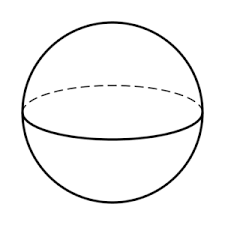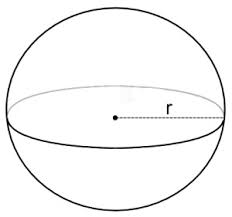## How to Calculate and Solve for the Volume and Radius of a Sphere | The Calculator EncyclopediaThe image above is a sphere.

To compute the volume of a sphere, one essential parameter is needed and this parameter is the radius of the sphere (r). You can also use diameter of the sphere (d).

The formula for calculating the volume of a sphere:

V = (4/3)πr³

Where;

V = Volume of the sphere
r = Radius of the sphere

Let’s solve an example:
Find the volume of a sphere when the radius of the sphere is 10 cm.

This implies that;

r = Radius of the sphere = 10 cm

V = (4/3)πr³
V = (4/3)π x (10)³
V = (4/3)π x 1000
V = (4/3)3.142 x 1000
V = (4/3)3142
V = 1.33 x 3142
V = 4188.7

Therefore, the volume of the sphere is 4188.7 cm³.

Calculating the Volume of a Sphere using Diameter of the Sphere.

V = 4πd³ / 24

Where;

V = Volume of the sphere
d = Diameter of the sphere

Let’s solve an example:
Find the volume of a sphere when the diameter of the sphere is 20 cm.

This implies that;

d = Diameter of the sphere = 20 cm

V = 4πd³ / 24
V = 4 x 3.142 x (20)³ / 24
V = 4 x 3.142 x 8000 / 24
V = 100544 / 24
V = 4189.3

Therefore, the volume of the sphere with diameter is 4189.3 cm3.

Calculating the Radius of a Sphere using Volume of the Sphere.

r = 3√(3V / )

Where;

V = Volume of the sphere
r = Radius of the sphere

Let’s solve an example:
Find the radius of a sphere when the volume of the sphere is 250 cm3.

This implies that;

V = Volume of the sphere = 250 cm3

r = 3√(3V / )
r = 3√(3 x 250 / 4 x 3.142)
r = 3√(750 / 12.568)
r = 3√59.675
r = 3.907

Therefore, the radius of the sphere is 3.907 cm.

Calculating the Diameter of a Sphere using Volume of the Sphere.

d = 3√(24V / )

Where;

A = Area of the sphere
d = Diameter of the sphere

Let’s solve an example:
Find the diameter of a sphere when the volume of the sphere is 40 cm3.

This implies that;

V = Volume of the sphere = 40 cm3

d = 3√(24V / )
d = 3√(24 x 40 / 4 x 3.142)
d = 3√(960 / 12.568)
d = 3√76.38
d = 4.24

Therefore, the diameter of the sphere is 4.24 cm.

## How to Calculate and Solve for the Area and Radius of a Sphere | Nickzom CalculatorThe image above is a sphere.

To compute the area of a sphere, one essential parameter is needed and this parameter is the radius of the sphere (r). You can also use diameter of the sphere (d).

The formula for calculating the area of a sphere:

A = 4πr²

Where;

A = Area of the sphere
r = Radius of the sphere

Let’s solve an example:
Find the area of a sphere when the radius of the sphere is 6 cm.

This implies that;

r = Radius of the sphere = 6 cm

A = 4πr²
A = 4 x 3.142 x 6²
A = 4 x 3.142 x 36
A = 452.4

Therefore, the area of the sphere is 452.4 cm2.

Calculating the Area of a sphere using Diameter of the sphere.

A = πd2

Where;

A = Area of the sphere
d = Diameter of the sphere

Let’s solve an example:
Find the area of a sphere when the diameter of the sphere is 8 cm.

This implies that;

d = Diameter of the sphere = 8 cm

A = πd2
A = 3.142 x 82
A = 3.142 x 64
A = 201.08

Therefore, the area of the sphere is 201.08 cm2.

Calculating the Radius of a sphere using Area of the sphere.

r = √(A / )

Where;

A = Area of the sphere
r = Radius of the sphere

Let’s solve an example:
Find the radius of a sphere when the area of the sphere is 22 cm2.

This implies that;

A = Area of the sphere = 22 cm2

r = √(A / )
r = √(22 / 4 x 3.142)
r = √(22 / 12.57)
r = √1.75
r = 1.32

Therefore, the radius of the sphere is 1.32 cm.